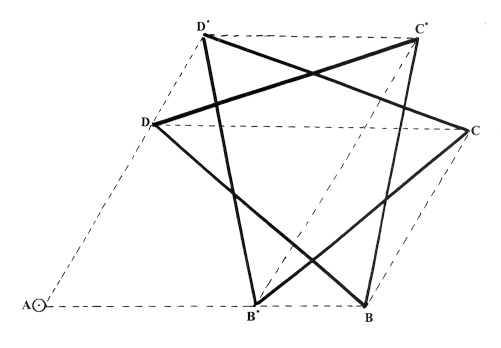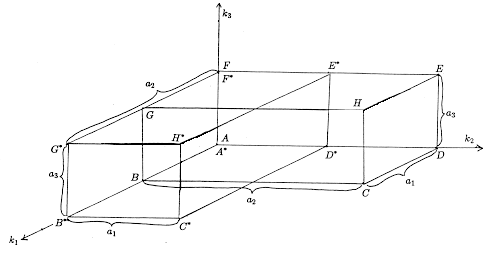## 0. Introduction

In a series of earlier papers [2, 3, 4, 5], the authors, and their collaborators, studied various patterns in the Pascal Triangle of binomial coefficients; and extended the Pascal Triangle, and the patterns, to encompass negative integral values of n and r in the binomial coefficient

 æ ç è n r ö ÷ ø

The resulting extension of the Pascal Triangle was described as the Pascal Hexagon, the central portion of which appears in Figure 1(a).

In those earlier papers the following pattern figured prominently: given a 'fundamental parallelogram' P = ABCDin the Pascal Triangle with sides in the coordinate directions n=constant, r=constant (see Figure 1(b)), and vertices

 A = æ ç è n r ö ÷ ø ,     B = æ ç è n r+l ö ÷ ø ,
 C = æ ç è n-k r+l ö ÷ ø ,     D = æ ç è n-k r ö ÷ ø

one calculates the weight

 W(P) = A×C B×D

and discovers it is invariant under the action of sliding the parallelogram in the direction n-r=constant. It is also invariant under the involution I that exchanges the roles of k and l. Notice that the vertex A, which we describe as the anchor of the parallelogram, is fixed under I.

Let P*=AB*C*D* be the image of P under I. Then, since

 W(P*) = A×C* B*×D*
and W(P)=W(P*), we see that
 B*×C×D* = B×C*×D
(0.1)

Figure 2 should make it plain why we have described (0.1) as a Star of David Theorem. It is very substantially generalized from the original special case , in which no distortion of the star takes place.Figure 2.

The pattern becomes even clearer if we adopt the full binomial notation

 æ ç è n r     s ö ÷ ø

with r+s=n, for the binomial coefficient. For then it is natural to expect - and easy to prove - that there are, in fact, three such patterns, in which the sides of the fundamental parallelogram are in two of the coordinate directions n=constant, r=constant, s=constant, and sliding takes place in the third direction. One need not even confine the action to the Pascal Triangle; these patterns also hold in the Pascal Hexagon. Thus a fundamental parallelogram may be slid across a 'zero' region (where, of course, the weight takes the form 0/0) into another non-zero region and recover the original weight in the new region.

In this paper we are concerned with generalizing these ideas to the multinomial coefficients

æ
ç
è
 n
 k1
 k2
 ...
 km
ö
÷
ø
Let us first consider the case m=3 so that trinomial coefficients are in question. Anticipating the more general case, we will write
æ
ç
è
 n
 k1
 k2
 k3
ö
÷
ø
 æ ç è n r     s     t ö ÷ ø
which features in Figures
5, 6, and 7 next we consider a fundamental parallelepiped P, with vertices

A    C   E   G
B   D   F   H

where
æ
ç
è
 n
 k1
 k2
 k3
ö
÷
ø
,    C æ
ç
è
 n+a1+a2
 k1+a1
 k2+a2
 k3
ö
÷
ø
,
æ
ç
è
 n+a2+a3
 k1
 k2+a2
 k3+a3
ö
÷
ø
,    G æ
ç
è
 n+a1+a3
 k1+a1
 k2
 k3+a3
ö
÷
ø
,
æ
ç
è
 n+a1
 k1+a1
 k2
 k3
ö
÷
ø
,     D æ
ç
è
 n+a2
 k1
 k2+a2
 k3
ö
÷
ø
,
F æ
ç
è
 n+a3
 k1
 k2
 k3+a3
ö
÷
ø
,     H æ
ç
è
 n+a1+a2+a3
 k1+a1
 k2+a2
 k3+a3
ö
÷
ø
(0.2)

We describe the vertices A, C, E, G, as the even vertices, and B, D, F, H as the odd vertices, because the number of different edges traversed between the anchor A and the vertex in question (regardless of the length of the edge) is an even number for A, C, E, G, and an odd number for B, D, F, H. This fact may be seen by counting the number of edges between the anchor and the vertex, on the illustration in Figure 3. Equivalently, the parity may be determined by counting the number of ki's that are changed in the bottom of the symbol

æ
ç
è
 n
 k1
 k2
 k3
ö
÷
ø
This method will, of course, be useful when we generalize to higher dimensions.Figure 3.

The weight of P is given by

 W(P) = product     of     even     vertices product    of     odd     vertices = A×C×E×G B×D×F×H ,

so that, by a straightforward calculation,
 W(P) = n!(n+a1+a2)!(n+a2+a3)!(n+a1+a3)! (n+a1)!(n+a2)!(n+a3)!(n+a1+a2+a3)! (0.3)

Thus, for fixed a1, a2, a3, W(P) is independent of k1, k2, k3, and invariant under the action of sliding P in the plane n=constant. Further, the symmetric group S3 acts on the triple (a1, a2, a3). For any p ÎS3 let P* be the p-image of P, with its vertices labelled in the obvious way. Then A is invariant under p and we get a Hyperstar of David Theorem of the form

 B*×C×D*×E×F*×G×H* = B×C*×D×E*×F×G*×H      (0.4)

If p is a cyclic permutation of length 3 (as illustrated in Figure 3), then (0.4) is a genuinely new identity. However, if p leaves some aj fixed, then (0.4) degenerates to a disguised form of (0.1). Thus if p simply exchanges a1 and a2, then p leaves both the anchor A and the vertex F fixed (F*=F), and (0.4) is a simple consequence of the two Star of David identities

 B×C*×D = B*×C×D*
 E×G×H* = E*×G*×H       (0.5)
at 'levels' k3 and k3+a3, respectively (see Figure 4).Figure 4.

Our other concern is to extend the domain of the multinomial coefficients

æ
ç
è
 n
 k1
 k2
 ...
 km
ö
÷
ø
to permit any integer values of n, k1, k2, ..., km, subject, of course, to the identity n=k1+k2+... +km.

The case m=3 is then of special geometrical interest. In this case it is natural to ask "What is the 3-dimensional analogue of the Pascal Hexagon?" We certainly expect the analogue of the Pascal Triangle to be the Pascal Tetrahedron (see Figure 5). In fact, extending the Pascal Tetrahedron in 3-dimensions, in a manner analogous to extending the Pascal Triangle in 2-dimensions, produces a certain semi-regular (Archimedean) polyhedron. Here we are content briefly to identify it as the cuboctahedron, but in an Appendix to this paper we give the complete answer to our question along with many features of the analogy.

A useful piece of information concerning this polyhedron is found in Section 2; namely, for general m, if n is negative, then

æ
ç
è
 n
 k1
 k2
 ...
 km
ö
÷
ø
will be non-zero if and only if exactly one of the ki is negative. If, for example, k1<0, then, in fact,
æ
ç
è
 n
 k1
 k2
 ...
 km
ö
÷
ø
= (-1)n-k1 æ
ç
è
 -k1-1
 -n-1
 k2
 ...
 km
ö
÷
ø
(0.6)

These (generalized) multinomial coefficients may then be used to provide power series expansions of (a1+a2+... +am)n, for n<0 in suitable domains of Rm.

In Section 1 we describe the properties of weights of fundamental hyperparallelepipeds (hppd's) in dimension m. We formulate and establish a general Hyperstar of David Theorem. In Section 2 we extend the Pascal m-simplex of multinomial coefficients to allow n to be a negative integer, and justify formula (0.6). In Section 3 we discuss generalizations of our results. In particular we point out that the multinomial coefficients may be replaced by suitable separable functions of the variables n, k1, k2,...,km. We are also concerned in this section with generalizing an interesting observation of Hoggatt-Alexanderson  relating to the 'nearest neighbours' of a given multinomial coefficient

æ
ç
è
 n
 k1
 k2
 ...
 km
ö
÷
ø
Finally, in the Appendix, we answer the question above involving the possible physical arrangement of trinomial coefficients in space.